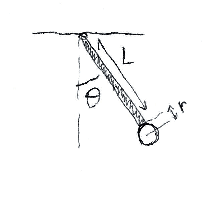# Small oscillation frequency of rod and disk pendulum

## Homework Statement

Consider a rod of length ##L## and mass ##M## attached on one end to the ceiling and on the other end to the edge of a disk of radius ##r## and mass ##m##. This system is slightly moved away from the vertical and let go. Let ##\theta## be the angle the pendulum makes with the vertical. What is the frequency ##\omega## of small oscillations of this pendulum?## Homework Equations

Newton's rotation law gives
$$\vec{\tau}=I\ddot{\theta}$$

where ##I## is the moment of inertia of the rod and disk relative to the pivot in the ceiling and ##\vec{\tau}## is the torque due to the gravity force.

## The Attempt at a Solution

[/B]
The torque due to gravity around the pivot ##P## divides into the torque of gravity at the center of mass of the rod (located at ##L/2##) plus the torque of the gravity force at the center of mass of the disk (located at ##L+r##). This is

$$\vec{\tau}=\vec{r}_{P,M}\times M\vec{g}+\vec{R}_{P,cm}\times m\vec{g}$$

This ends up giving

$$\vec{\tau}=-\left[M\frac{L}{2} +m(L+r)\right]g\sin\theta\hat{z}$$

So the equation of motion $$\vec{\tau}=I\ddot{\theta}$$ becomes

$$-\left[M\frac{L}{2} +m(L+r)\right]g\sin\theta=I\ddot{\theta}$$

If we make this an harmonic type equation where ##\ddot{\theta}=-\omega^{2}\theta,\sin\theta\approx \theta ## it becomes ##-\left[M\frac{L}{2} +m(L+r)\right]g\theta=-I\omega^{2}\theta##

and so

$$\omega=\sqrt{\frac{\left[M\frac{L}{2} +m(L+r)\right]g}{I}}$$

This is not the solution for the frequency of oscillation, which means I am missing something perhaps in the center of mass calculation.

#### Attachments

Last edited:

TSny
Homework Helper
Gold Member
Your result looks OK to me. In what way does your answer differ from the solution that you were given?

It looks like you have a typographical error where you left out a factor of ##\theta## on the right side of
##-\left[M\frac{L}{2} +m(L+r)\right]g\theta=-I\omega^{2}##
But this doesn't affect your result.

Your result looks OK to me. In what way does your answer differ from the solution that you were given?
The solution given is

$$\omega=\sqrt{\frac{\left[M\frac{L}{2}+m(L+r)\right]gM}{I(M+m)}}$$

TSny
Homework Helper
Gold Member
To see which answer cannot be correct, try a special case where you already know the answer. For example, suppose you transform the pendulum into a simple pendulum by letting the mass of the rod equal zero and also letting the radius of the disk equal zero. So, you would have a point mass m at the end of a massless rod of length L.

•Jenny Physics
To see which answer cannot be correct, try a special case where you already know the answer. For example, suppose you transform the pendulum into a simple pendulum by letting the mass of the rod equal zero and also letting the radius of the disk equal zero. So, you would have a point mass m at the end of a massless rod of length L.
In that case my solution would give ##\omega=\sqrt{g/L}## while the official solution would give ##\omega=0## so my solution would seem to win out.

TSny
Homework Helper
Gold Member
In that case my solution would give ##\omega=\sqrt{g/L}## while the official solution would give ##\omega=0## so my solution would seem to win out.
Absolutely.